# Multiply a 3-digit number by a 1-digit number

## Learning focus

Learn how to multiply hundreds, tens and ones by ones.

This includes:

• a quiz
• a learning summary

# Quiz

To get started, let's see how well you know this topic already. Take the catch-up quiz below to find out.

# Learn

## Short multiplication

Partition the number into ones, tens and hundreds. Multiply these by the 1-digit number separately. Make sure everything is in the right place value column.

## What is 342 × 4?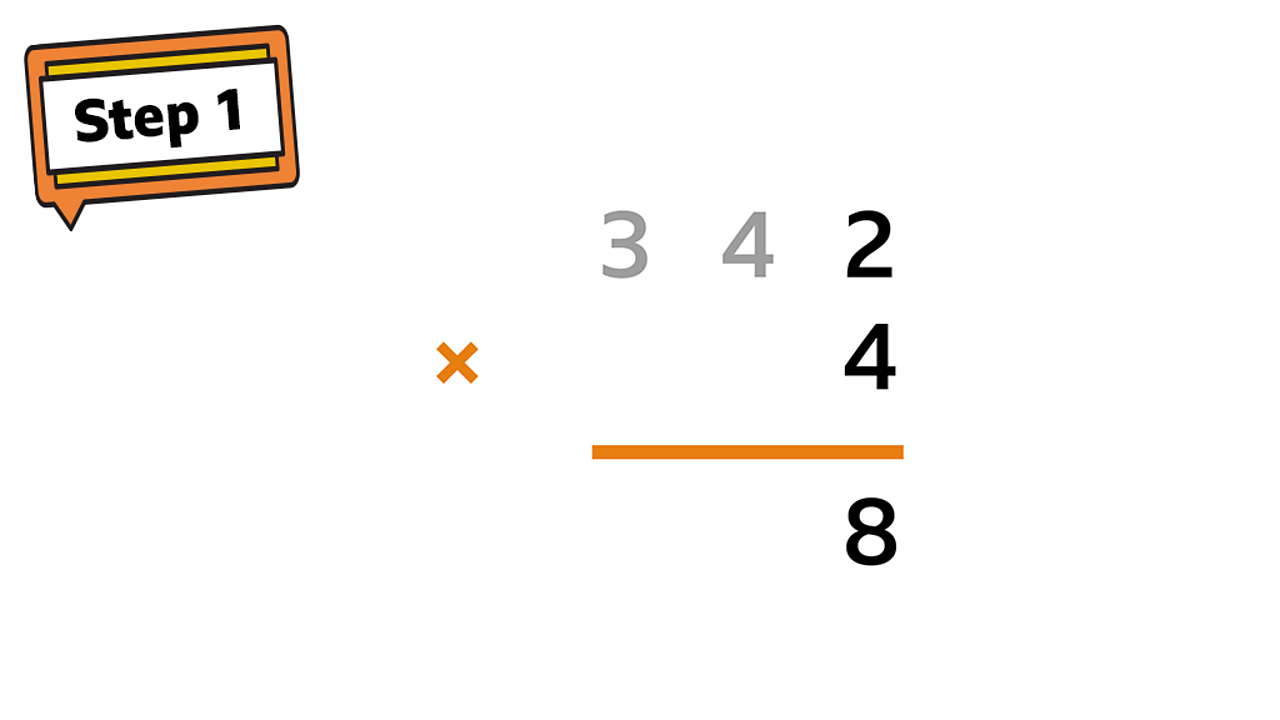Start by multiplying the digit in the ones by 4. 2 × 4 = 8.1 of 4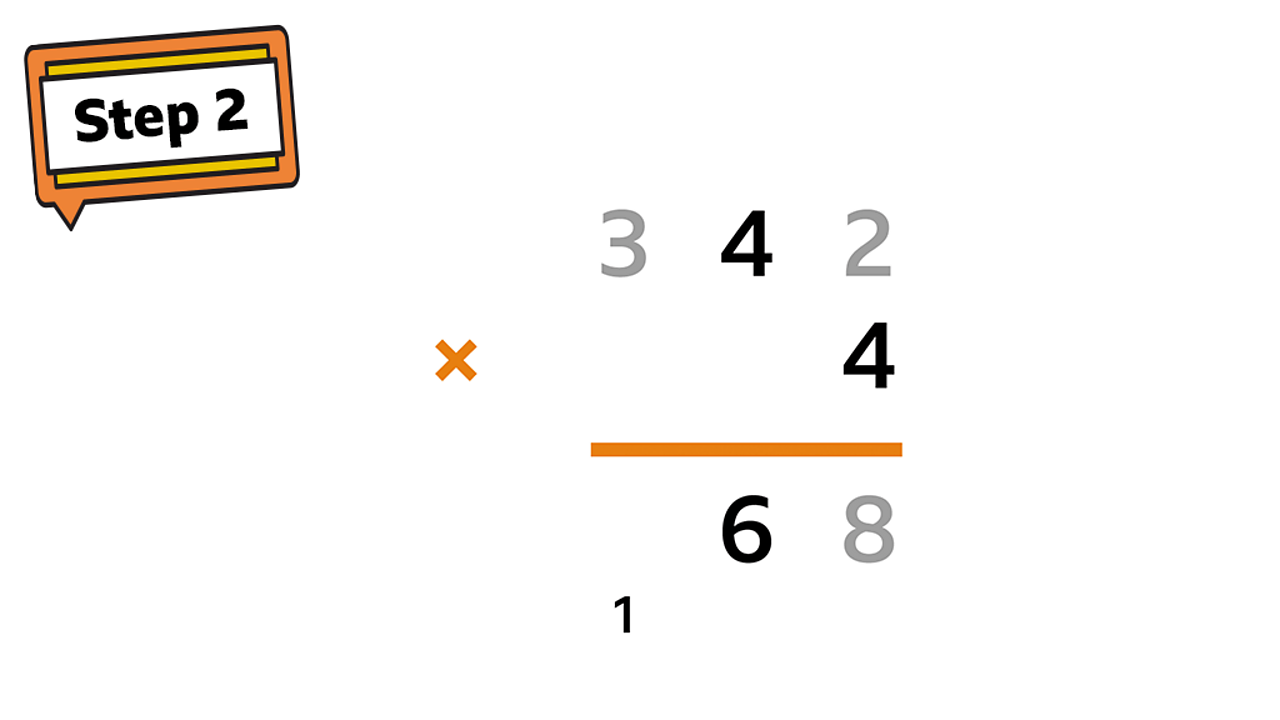Now multiply the tens by 4. 40 × 4 = 160. Place the 6 tens in the tens column and carry the hundred over into the hundreds column.2 of 4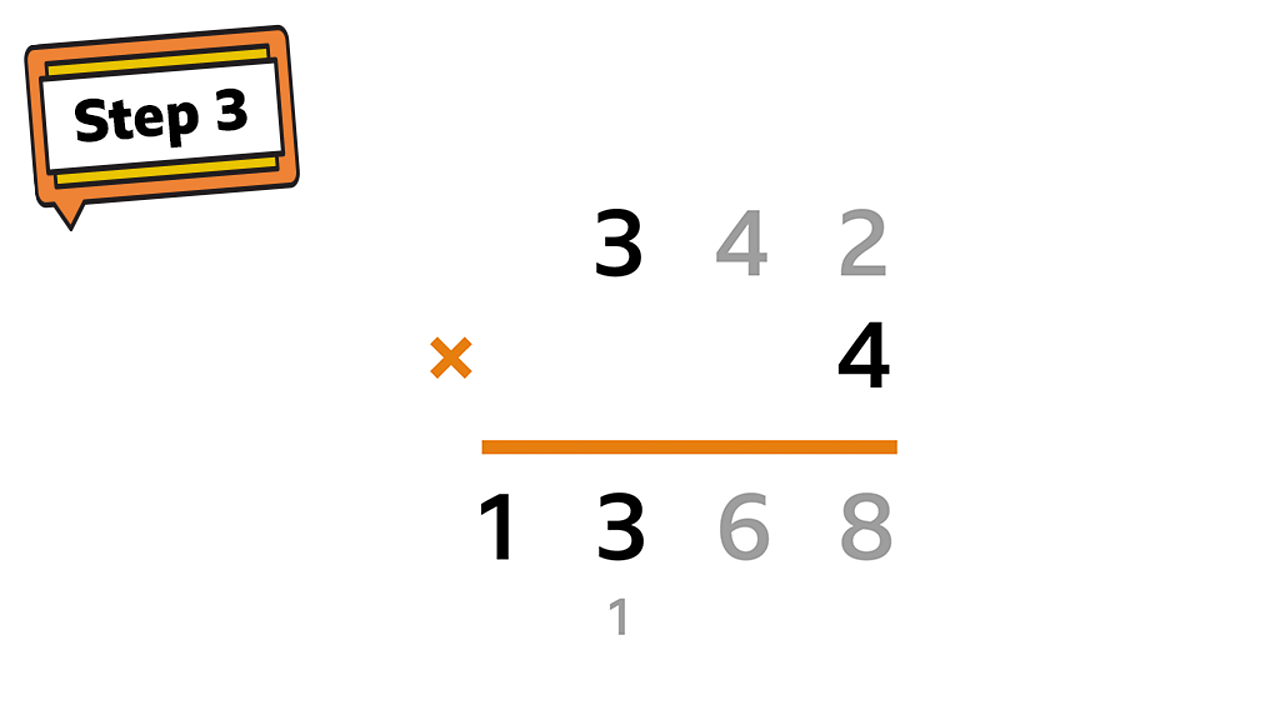Multiply 300 by 4. 300 × 4 = 1200. Don’t forget to add the hundred you carried over! 1200 + 100 = 1300. Place the 3 hundreds in the hundreds column and the 1 in the thousands column.3 of 4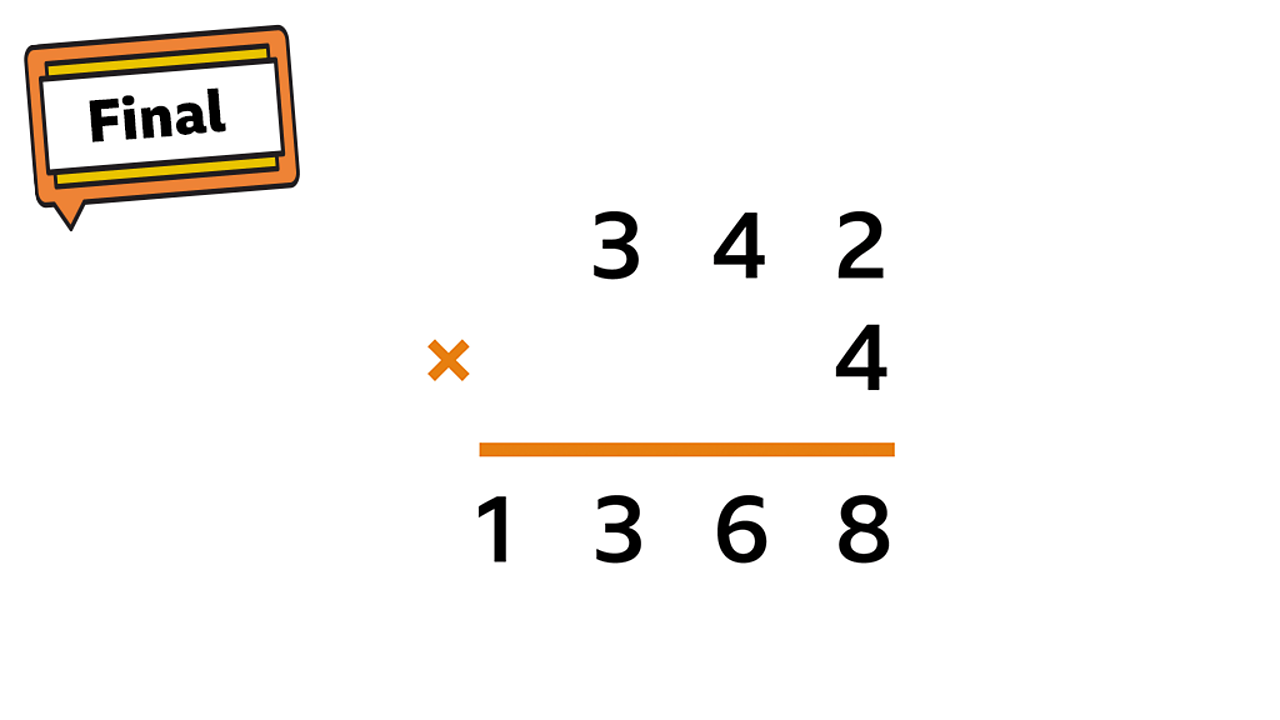Now you have solved it: 342 × 4 = 1368.4 of 4

## What is 317 × 5?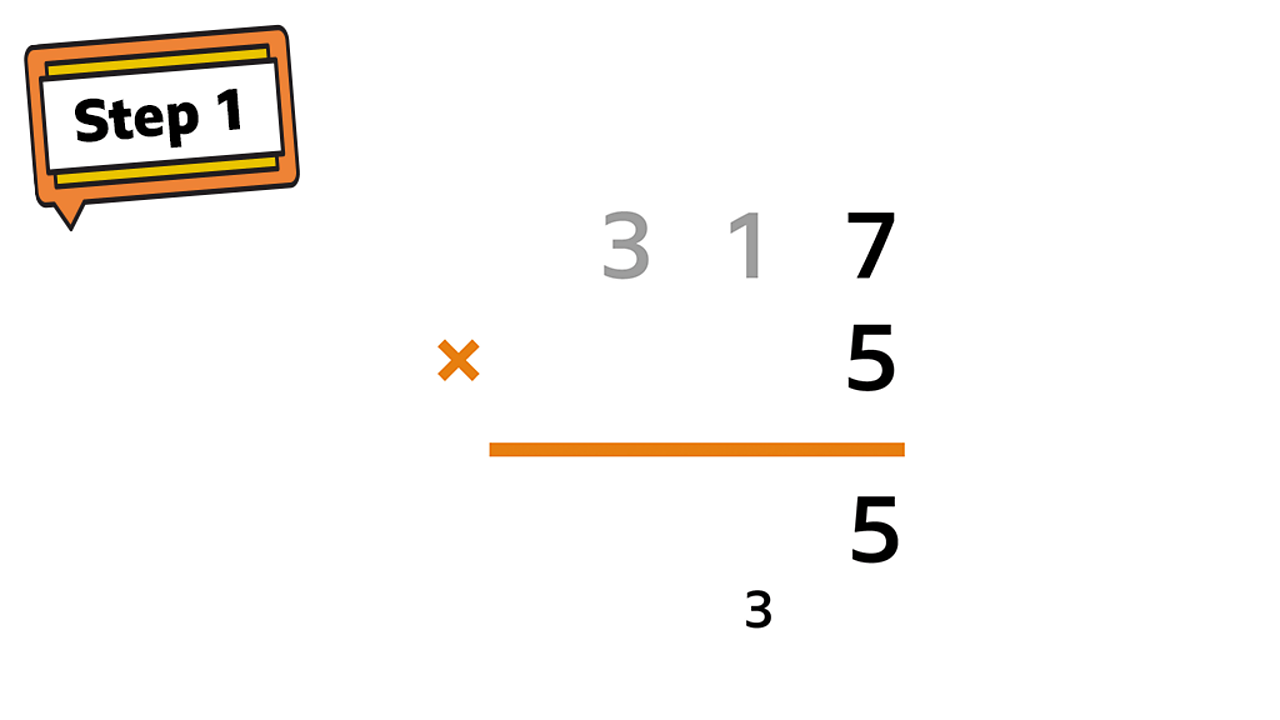Multiply the ones by 5. 7 × 5 = 35. Keep the 5 in the ones column and carry over the 3 into the tens.1 of 4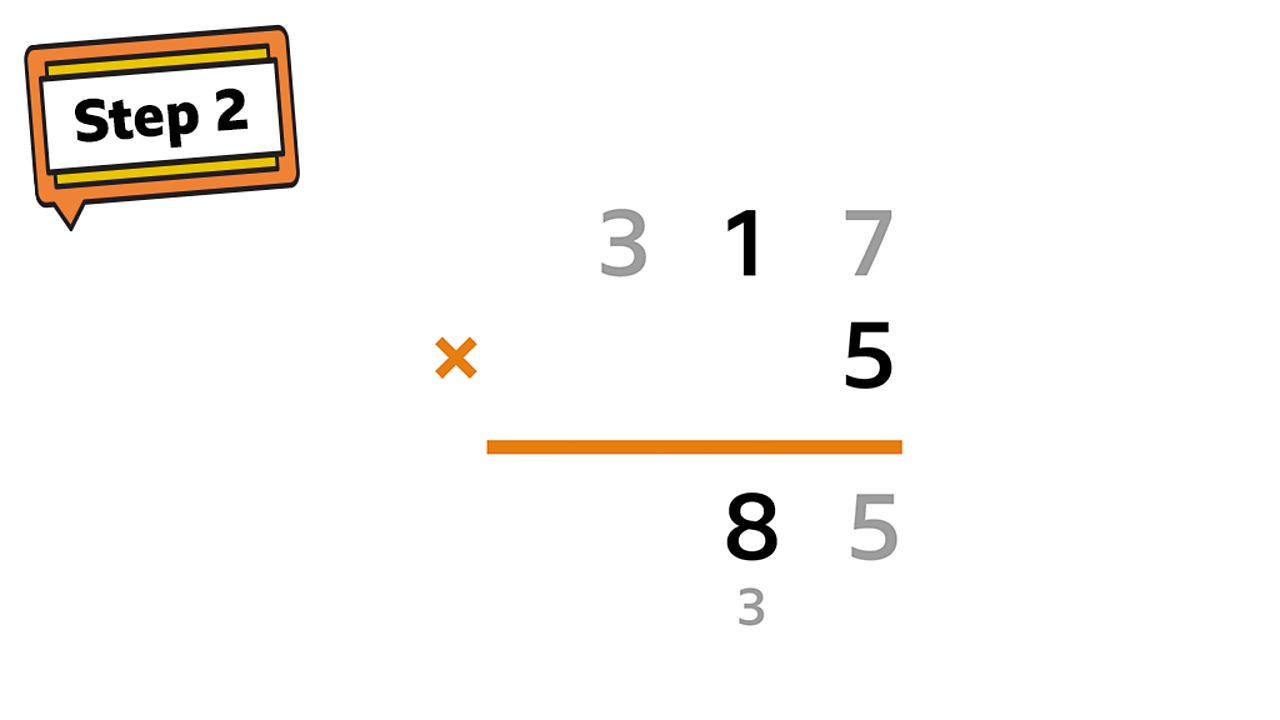Now multiply the tens by 5. 10 × 5 = 50. Add on the 3 extra tens that you carried over before. 50 + 30 = 80.2 of 4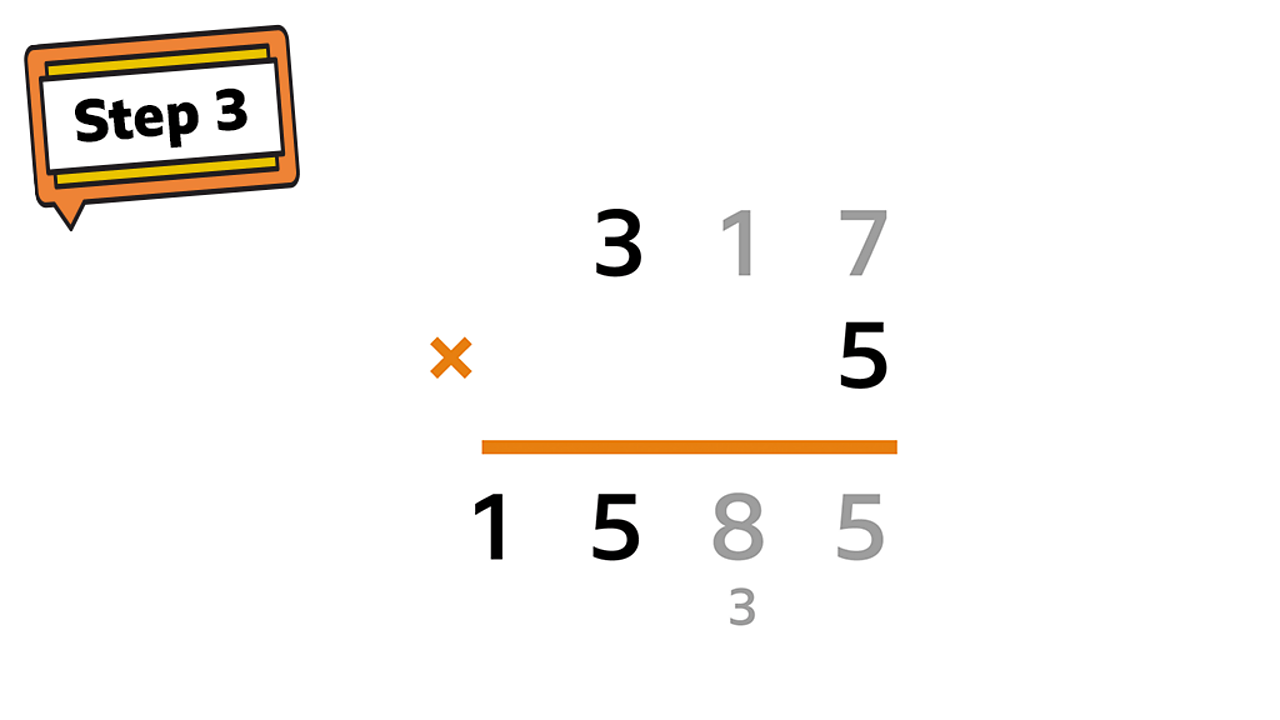Multiply 300 by 5. 300 × 5 = 1500.3 of 4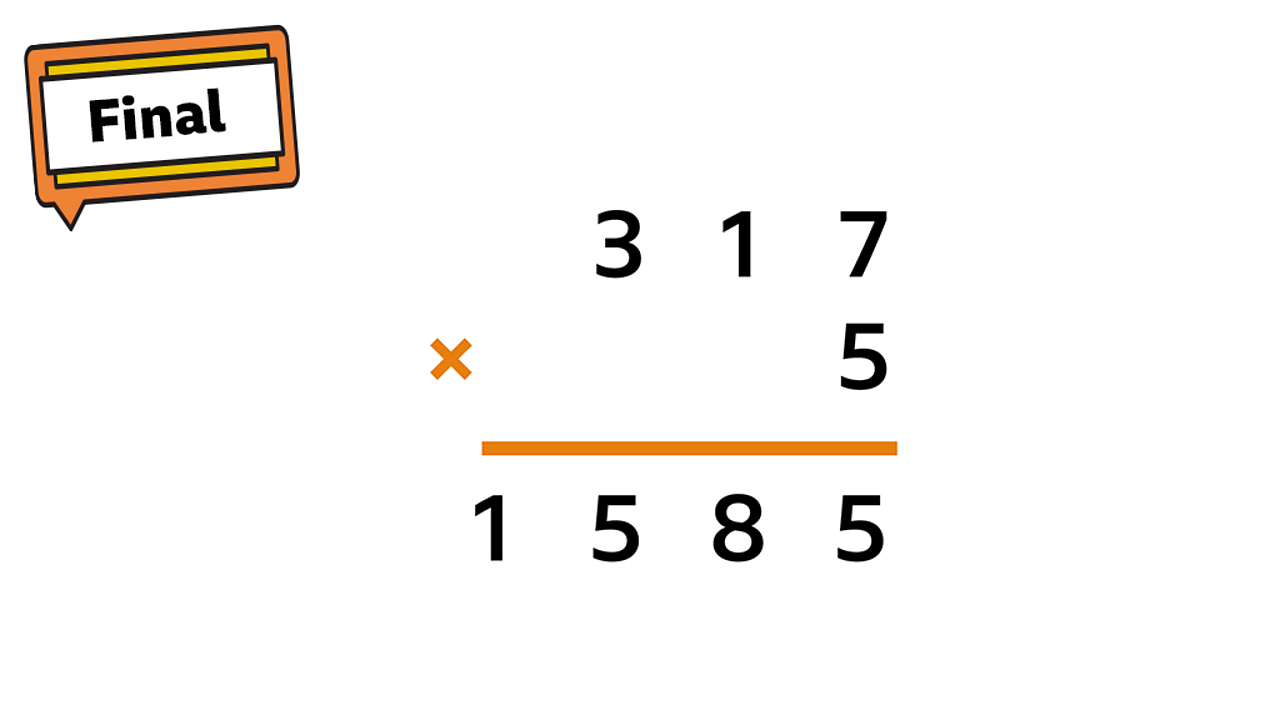You have now solved it! 317 × 5 = 1585.4 of 4

When following the short multiplication method, always double check your working out and that each digit is in the correct place.